JMSLTM Numerical Library 7.2.0
com.imsl.datamining.neural

Class ScaleFilter

• All Implemented Interfaces:
Serializable

public class ScaleFilter
extends Object
implements Serializable
Scales or unscales continuous data prior to its use in neural network training, testing, or forecasting.

Bounded scaling is used to ensure that the values in the scaled array fall between a lower and upper bound. The scale limits have the following interpretation:

 Argument Interpretation realMin The lowest value expected in x. realMax The largest value expected in x. targetMin The lower bound for the values in the scaled data. targetMax The upper bound for the values in the scaled data.

The scale limits are set using the method setBounds.

The specific scaling used is controlled by the argument scalingMethod used when constructing the filter object. If scalingMethod is NO_SCALING, then no scaling is performed on the data.

If the scalingMethod is BOUNDED_SCALING then the bounded method of scaling and unscaling is applied to x. The scaling operation is conducted using the scale limits set in method setBounds, using the following calculation: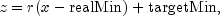where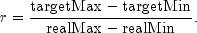If scalingMethod is one of UNBOUNDED_Z_SCORE_SCALING_MEAN_STDEV, UNBOUNDED_Z_SCORE_SCALING_MEDIAN_MAD, BOUNDED_Z_SCORE_SCALING_MEAN_STDEV, or BOUNDED_Z_SCORE_SCALING_MEDIAN_MAD, then the z-score method of scaling is used. These calculations are based upon the following scaling calculation: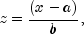where a is a measure of center for x, and b is a measure of the spread of x.

If scalingMethod is UNBOUNDED_Z_SCORE_SCALING_MEAN_STDEV, or BOUNDED_Z_SCORE_SCALING_MEAN_STDEV, then a and b are the arithmetic average and sample standard deviation of the training data.

If scalingMethod is UNBOUNDED_Z_SCORE_SCALING_MEDIAN_MAD or BOUNDED_Z_SCORE_SCALING_MEDIAN_MAD, then a and b are the median and, whereis a robust estimate of the population standard deviation: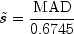where MAD is the Mean Absolute Deviation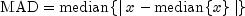The Mean Absolute Deviation is a robust measure of spread calculated by finding the median of the absolute value of differences between each non-missing value for the ith variable and the median of those values.

If the method decode is called then an unscaling operation is conducted by inverting using: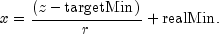Unbounded z-score Scaling

If scalingMethod is UNBOUNDED_Z_SCORE_SCALING_MEAN_STDEV or UNBOUNDED_Z_SCORE_SCALING_MEDIAN_MAD, then a scaling operation is conducted using the z-score calculation: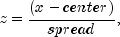If scalingMethod is UNBOUNDED_Z_SCORE_SCALING_MEAN_STDEV then center is set equal to the arithmetic average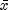of x, and spread is set equal to the sample standard deviation of x. If scalingMethod is UNBOUNDED_Z_SCORE_SCALING_MEDIAN_MAD then center is set equal to the median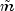of x, and spread is set equal to the Mean Absolute Difference (MAD).

The method decode can be used to unfilter data using the the inverse calculation for the above equation: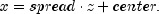Bounded z-score Scaling

This method is essentially the same as the z-score calculation described above with additional scaling or unscaling using the scale limits set in method setBounds. The scaling operation is conducted using the well known z-score calculation: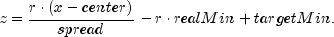If scalingMethod is UNBOUNDED_Z_SCORE_SCALING_MEAN_STDEV then center is set equal to the arithmetic averageof x, and spread is set equal to the sample standard deviation of x. If scalingMethod is UNBOUNDED_Z_SCORE_SCALING_MEDIAN_MAD then center is set equal to the medianof x, and spread is set equal to the Mean Absolute Difference (MAD).

The method decode can be used to unfilter data using the the inverse calculation for the above equation: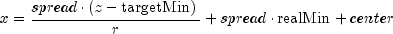Example, Serialized Form
• Constructor Summary

Constructors
Constructor and Description
ScaleFilter(int scalingMethod)
Constructor for ScaleFilter.
• Method Summary

Methods
Modifier and Type Method and Description
double decode(double z)
Unscales a value.
double[] decode(double[] z)
Unscales an array of values.
void decode(int columnIndex, double[][] z)
Unscales a single column of a two dimensional array of values.
double encode(double x)
Scales a value.
double[] encode(double[] x)
Scales an array of values.
void encode(int columnIndex, double[][] x)
Scales a single column of a two dimensional array of values.
double[] getBounds()
Retrieves bounds used during bounded scaling.
double getCenter()
Retrieves the measure of center to be used during z-score scaling.
Retrieves the measure of spread to be used during scaling.
void setBounds(double realMin, double realMax, double targetMin, double targetMax)
Sets bounds to be used during bounded scaling and unscaling.
void setCenter(double center)
Set the measure of center to be used during z-score scaling.
Set the measure of spread to be used during z-score scaling.
• Field Detail

• BOUNDED_SCALING

public static final int BOUNDED_SCALING
Flag to indicate bounded scaling.
Constant Field Values
• BOUNDED_Z_SCORE_SCALING_MEAN_STDEV

public static final int BOUNDED_Z_SCORE_SCALING_MEAN_STDEV
Flag to indicate bounded z-score scaling using the mean and standard deviation.
Constant Field Values

Flag to indicate bounded z-score scaling using the median and mean absolute difference.
Constant Field Values
• NO_SCALING

public static final int NO_SCALING
Flag to indicate no scaling.
Constant Field Values
• UNBOUNDED_Z_SCORE_SCALING_MEAN_STDEV

public static final int UNBOUNDED_Z_SCORE_SCALING_MEAN_STDEV
Flag to indicate unbounded z-score scaling using the mean and standard deviation.
Constant Field Values

Flag to indicate unbounded z-score scaling using the median and mean absolute difference.
Constant Field Values
• Method Detail

• decode

public double decode(double z)
Unscales a value.
Parameters:
z - A double containing the value to be unscaled.
Returns:
A double containing the filtered data.
• decode

public double[] decode(double[] z)
Unscales an array of values.
Parameters:
z - A double array of values to be unscaled.
Returns:
A double array containing the filtered data.
• decode

public void decode(int columnIndex,
double[][] z)
Unscales a single column of a two dimensional array of values.
Parameters:
columnIndex - An int specifying the index of the column of z to unscale. Indexing is zero-based.
z - A double matrix containing the values to be unscaled. Its columnIndex-th column is modified in place.
• encode

public double encode(double x)
Scales a value.
Parameters:
x - A double containing the value to be scaled.
Returns:
A double containing the scaled value.
• encode

public double[] encode(double[] x)
Scales an array of values.
Parameters:
x - A double array containing the data to be scaled.
Returns:
A double array containing the scaled data.
• encode

public void encode(int columnIndex,
double[][] x)
Scales a single column of a two dimensional array of values.
Parameters:
columnIndex - An int specifying the index of the column of x to scale. Indexing is zero-based.
x - A double matrix containing the value to be scaled. Its columnIndex-th column is modified in place.
• getBounds

public double[] getBounds()
Retrieves bounds used during bounded scaling.
Returns:
A double array of length 4 containing the values

 i result[i] 0 realMin. Lowest expected value in the data to be filtered. 1 realMax. Largest expected value in the data to be filtered. 2 targetMin. Lowest allowed value in the filtered data. 3 targetMax. Largest allowed value in the filtered data.

• getCenter

public double getCenter()
Retrieves the measure of center to be used during z-score scaling.
Returns:
A double containing the measure of center to be used during z-score scaling.

Retrieves the measure of spread to be used during scaling.
Returns:
a double containing the measure of spread to be used during scaling.
• setBounds

public void setBounds(double realMin,
double realMax,
double targetMin,
double targetMax)
Sets bounds to be used during bounded scaling and unscaling. This method is normally called prior to calls to encode or decode. Otherwise the default bounds are realMin = 0, realMax = 1, targetMin = 0, and targetMax = 1. These bounds are ignored for unbounded scaling.
Parameters:
realMin - A double containing the lowest expected value in the data to be filtered.
realMax - A double containing the largest expected value in the data to be filtered.
targetMin - A double containing the lowest allowed value in the filtered data.
targetMax - A double containing the largest allowed value in the filtered data.
• setCenter

public void setCenter(double center)
Set the measure of center to be used during z-score scaling.
Parameters:
center - A double containing the measure of center to be used during scaling. If this method is not called then the measure of center is computed from the data.